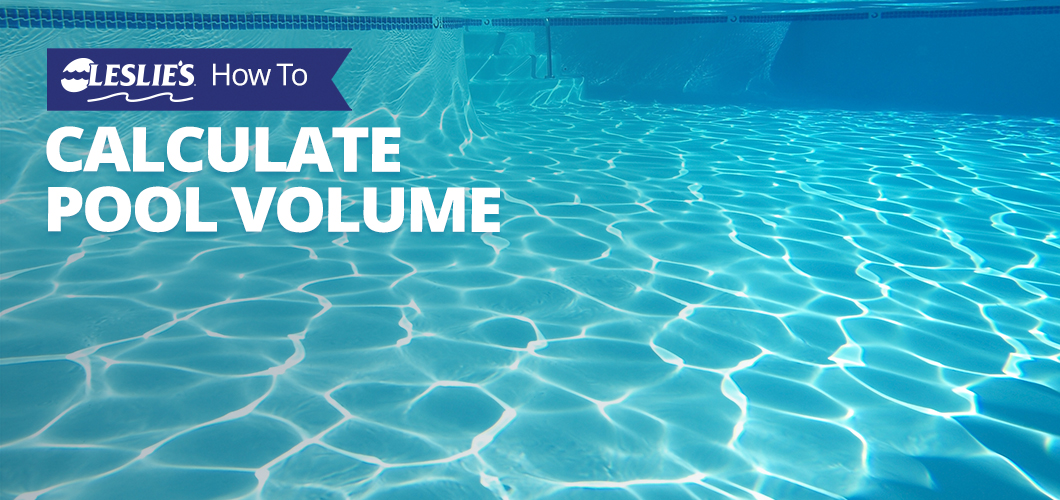# How To Calculate Pool Volume

Like a cook who needs to know how much of each ingredient to add to a dish, pool owners need to know how much of their maintenance products they need to add to their water. In order to do this properly, pool owners must know their pool volume — how much water is actually in their pool.

Calculating your pool volume is essential to correctly balancing the water. Making only rough estimates can result in errors and end up causing issues. Over-estimating is a waste of money; under-estimating will result in unsanitary water. If the volume of your pool isn't in the original documentation, it's easy to calculate it yourself.

## Running The Numbers

Three average measurements are used to calculate pool volume for most pools: length, width, and depth. There are more complicated formulas for measuring oddly-shaped pools, but most of them also include these measurements.

If your pool has a shallow end and a deep end, you'll need to measure both in order to find the average depth. For example: The shallow end is 3' deep and the deep end is 8'. Using the Average Depth formula: (3+8)÷2=5.5' Average Depth.)

As you'll notice below, most of these formulas are multiplied by 7.5, which is approximately how many gallons are in a cubic foot. The pool size measurements will help you find the cubic footage of the pool, and multiplying this by 7.5 will help you find a close estimate of volume in gallons.

Once you have this number, you should be able to apply the correct amount of pool chemicals to your pool water and avoid any future dosage issues.

The combined formulas should look like this:

Square and Rectangle Pools:

Length x Width x Average Depth x 7.5 = Approximate Volume (Gallons)

Round Pools:

3.14 x Radius x Radius x Average Depth x 7.5 = Volume (Gallons)

The Radius equals the diameter divided by 2. (Example: A 16' diameter divided by 2 = 8' radius.)

Note: Most round pools have a single depth.

Oval Pools:

3.14 x Length x Width x .25 x Average Depth x 7.5 = Approximate Volume (Gallons)

Kidney-Shaped Pool:

(A + B) x Length x 0.45 x Average Depth x 7.5 = Approximate Volume (Gallons)

Note: “A” and “B” measurements are the two widest points of the kidney shape.

Irregular Shapes or Free-Form Pools:

Longest Length x Widest Width x Average Depth x 5.9 = Approximate Volume (Gallons)

## Quick Reference

For your convenience, you can also consult the following charts. They detail the volume of many popular pool sizes.

### In-Ground Pool Volume (Standard Depth)

For more information on pool size or chemical usage, check our complete blog library, or reach out to one of our knowledgeable staff members at your local Leslie's. At Leslie's, your pool is our passion.